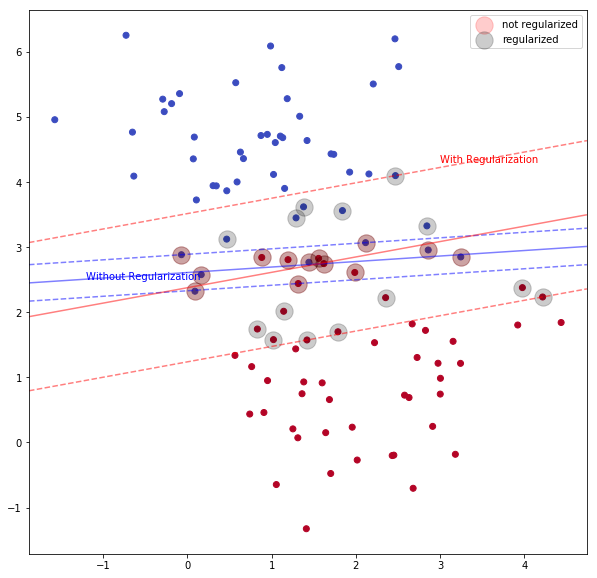# Support Vector Machine Part 1¶

### Elan Ding¶

Modified: July 24, 2018 $\newcommand{\bs}{\boldsymbol}$ $\newcommand{\argmin}{\underset{\bs{#1}}{\text{arg min}}\,}$ $\newcommand{\argmax}{\underset{\bs{#1}}{\text{arg max}}\,}$ $\newcommand{\tr}{^{\top}}$ $\newcommand{\norm}{\left|\left|\,#1\,\right|\right|}$ $\newcommand{\given}{\,|\,}$

I have always wanted to write a post about the support vector machine (SVM), so today I have decided to take a step back from the fascinating deep learning topics, and write about something that used to be a very hot topic before the deep learning era, and is still considered one of the best off-the-shelf classification algorithm today. SVM is a concept that is intuitive to learn but difficult to master. There are many literatures written about SVM: some are too basic, while some are overly complicated. So I would like to offer my own understanding of this amazing gem. The reason that SVM is hard to master is that one needs knowledge in not only statistics, but also linear algebra, and convex optimization. I am super excited, so let's get started.

## SVM in Separable Space¶

Given a set of data points, unlike traditional statistical methods, the SVM is taking a direct approach by asking a simple question:

Can we find a hyperplane that separate the classes in the feature space?

First let's assume that the feature space is separable. That is, we can always find a decision boundary that perfectly separate the classes. For example, we can generate a separable dataset using make_blobs. From earlier posts, I have shown two ways to find such decision boundary. One is using Gaussian discriminant analysis, and another is using logistic regression. Here, we will be using support vector machine.

In :
import numpy as np
import matplotlib.pyplot as plt
from sklearn.datasets.samples_generator import make_blobs
from sklearn.svm import SVC
%matplotlib inline

plt.figure(figsize=[10, 10])

# gereate data
X, y = make_blobs(n_samples=100, centers=2, cluster_std = 0.5, n_features=2, random_state=0)
plt.scatter(X[:,0], X[:,1], c=y, cmap=plt.cm.coolwarm)

# fit SVM linear model, no regularization
clf = SVC(kernel='linear', C=1000).fit(X,y)

# obtain the current figure's info and limits
ax = plt.gca()
xlim = ax.get_xlim()
ylim = ax.get_ylim()

# create meshgrid and obtain the distance from each point to the decision boundary
xx = np.linspace(xlim, xlim, 30)
yy = np.linspace(ylim, ylim, 30)
YY, XX = np.meshgrid(yy, xx)
xy = np.vstack([XX.ravel(), YY.ravel()]).T
Z = clf.decision_function(xy).reshape(XX.shape)

# plot decision boundary and margins
ax.contour(XX, YY, Z, colors='b', levels=[-1, 0, 1], alpha=0.5, linestyles=['--', '-', '--'])

# plot support vectors
ax.scatter(clf.support_vectors_[:, 0], clf.support_vectors_[:, 1], s=100, linewidth=1, facecolors='g')

# annotations
ax.annotate('$y=1$', xy=(2.0, 4.5), size=20)
ax.annotate('$y=-1$', xy=(0.7, 0.8), size=20)
ax.annotate('Decision Boundary', xy=(1.1, 2.6))
ax.annotate('Support Vector', xy=(clf.support_vectors_[0,0], clf.support_vectors_[0,1]))
ax.annotate('Support Vector', xy=(clf.support_vectors_[1,0], clf.support_vectors_[1,1]))
ax.annotate('Support Vector', xy=(clf.support_vectors_[2,0], clf.support_vectors_[2,1]))

Out:
Text(1.46871,1.86947,'Support Vector')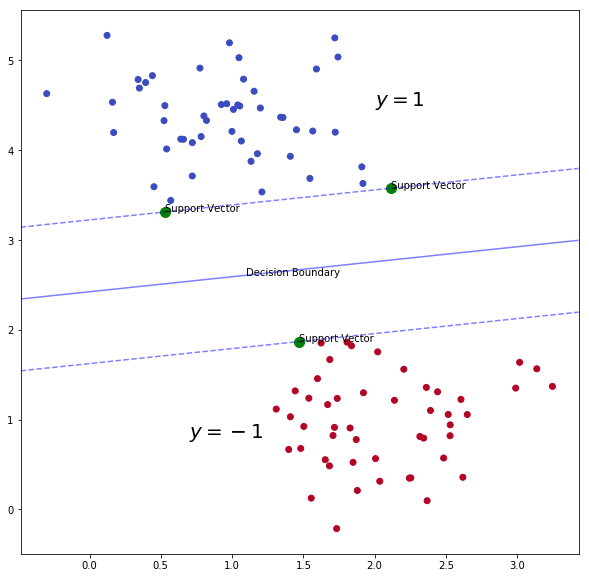The green dots in the plot are called support vectors. These are the points with the smallest geometric margin. In other words, they are closest to the decision boundary. Just by looking at the plot, we see that the support vectors are sufficient in finding the decision boundary!

Let's formalize the notion of geometric margin. Suppose that the decision boundary is found to be the line $\bs{w}\tr \bs{x}+b$, and $y \in \{1, -1\}$. Given a set of training examples $\{(\bs{x}^{(i)}, y^{(i)}) : i=1,...,m\}$, the geometric margin with respect to this set is defined as

$$\gamma = \min_{i} \frac{1}{\norm{\bs{w}}}y^{(i)}\left(\bs{w}\tr \bs{x}^{(i)} + b \right)$$

Intuitively, this is precisely the minimum distance from the points to the decision boundary. Suppose $b=0$, and $\bs{w}$ is a unit vector, then the term $\bs{w}\tr \bs{x}^{(i)}$ is simply the length of the the projection of $\bs{x}^{(i)}$ onto the direction orthogonal to the decision boundary. Suppose $\bs{x}^{(i)}$ is on the "positive" side of the decision boundary (in the picture, these are the blue points), then $y=1$ and $\bs{w}\tr \bs{x}^{(i)} \geq 0$. Hence $\gamma$ is precisely the distance from the point to the decision boundary. On the countrary, suppose $\bs{x}^{(i)}$ happens to be on the "negative" side of the decision boundary. Then $y=-1$ and $\bs{w}\tr \bs{x}^{(i)} <0$, so we still have a positive distance.

Given such a training set, our goal is to maximize the geometric margin. Hence, we need to solve the following optimization problem:

\begin{aligned} \max_{\gamma, \bs{w}, b} & \quad \gamma \\ \text{s.t.} & \quad \frac{1}{\norm{\bs{w}}}y^{(i)}(\bs{w}\tr \bs{x}^{(i)}+b) \geq \gamma, \,\, i=1,...,m \end{aligned}

This is equilvalent to

\begin{aligned} \max_{\gamma, \bs{w}, b} & \quad \gamma \\ \text{s.t.} & \quad y^{(i)}(\bs{w}\tr \bs{x}^{(i)}+b) \geq \norm{\bs{w}}\gamma, \,\, i=1,...,m \end{aligned}

Letting $\beta = \norm{\bs{w}} \gamma$, we can rewrite the problem as

\begin{aligned} \max_{\beta, \bs{w}, b} & \quad \frac{\beta}{\norm{\bs{w}}} \\ \text{s.t.} & \quad y^{(i)}(\bs{w}\tr \bs{x}^{(i)}+b) \geq \beta, \,\, i=1,...,m \end{aligned}

$\beta$ is also called the functional margin, which is not resistance to scaling of the parameters. (That is, we can scale $\bs{w}$ and $b$ and make the functional margin arbitrariliy large or small.) Using this property, we can scale our parameters in such ways so that $\beta=1$. Plugging this into our optimization problem, we see that the problem is equivalent to minimizing $\norm{\bs{w}}$. For mathematical convenience, we choose to solve the following primal optimization problem:

\begin{aligned} \min_{\bs{w}, b} & \quad \frac{1}{2}\norm{\bs{w}}^2 \\ \text{s.t.} & \quad y^{(i)}(\bs{w}\tr \bs{x}^{(i)}+b) \geq 1, \,\, i=1,...,m \end{aligned} \tag{1}

This problem can be easily solved using many off-the-counter optimization software, but before calling it quits, let's move a step further to discuss the kernels.

## The Kernel Trick¶

We can rewrite the constraint in (1) as $g_i(\bs{w}) = -y^{(i)}(\bs{w}\tr \bs{x}^{(i)} + b)+1 \leq 0$, we can construct the Lagrangian for our optimization problem as

$$\mathcal{L}(\bs{w}, b, \bs{\alpha}) = \frac{1}{2}\norm{\bs{w}}^2 - \sum_{i=1}^m \alpha_i \left[y^{(i)}(\bs{w}\tr \bs{w}^{(i)} +b)-1 \right]. \tag{2}$$

where $\bs{\alpha}$ is a vector of parameters called the Lagrange multipliers.

From the theory of convex optimization, given a primal optimization problem with the Lagrangian define above, we can find its corresponding dual problem as

$$\max_{\alpha_i \geq 0} \theta_D(\bs{\alpha}) = \max_{\alpha_i \geq 0} \min_{\bs{w, b}} \mathcal{L}(\bs{w}, b, \bs{\alpha}).$$

Furthermore, if there exists parameters $\bs{w}^*, b^*, \bs{\alpha}^*$ that satisfies the Karush-Kuhn-Tucker (KKT) conditions, which are

\begin{aligned} \nabla_{\bs{w}} \mathcal{L}(\bs{w}^*, b^* \bs{\alpha}^*) &= \bs{0}\\ \alpha_i^* g_i(\bs{w}^*) & = 0, i=1,...,m \\ g_i(\bs{w}^*) & \leq 0, i=1,...,m \\ \alpha_i^* & \geq 0, i=1,...,m \end{aligned} \tag{3}

then it is also a solution to both the primal and the dual problems.

In the process of deriving the dual problem of (2), one can show, with some algebra, that the dual problem indeed satisfies the KKT conditions. (I will skip the details here.) However, there is one essential detail that we cannot skip. Suppose that the optimal solution has been found to be $\bs{w}^*, b^*$ and $\bs{\alpha}^*$. Going back to the first step of finding $\theta_D(\bs{\alpha})$ by minimizing the Lagrangian, we have

$$\nabla_{\bs{w}} \mathcal{L}(\bs{w}^*, b^*, \bs{\alpha}^*) = \bs{w}^* - \sum_{i=1}^m \alpha_i^* y^{(i)} \bs{x}^{(i)} = 0$$

This simplies to

$$\bs{w}^* = \sum_{i=1}^m \alpha_i^* y^{(i)} \bs{x}^{(i)} \tag{4}$$

So once we have found the optimal values of the parameters, given any trainig example $\bs{x}$, if we want to make a decision about its corresponding label $y \in \{1, -1\}$, we can simply plug (4) into our decision function $\bs{w}\tr \bs{x} + b$:

\begin{aligned} {\bs{w}^*}\tr \bs{x} +b^* &= \left( \sum_{i=1}^m \alpha_i^* y^{(i)}\bs{x}^{(i)} \right)\tr \bs{x} +b^* \\ &= \sum_{i=1}^m \alpha_i^* y^{(i)} \left<\bs{x}^{(i)}, \bs{x}\right> +b^* \end{aligned}\tag{5}

and predict $y=1$ if the value is positivei and $y=-1$ if the value of negative. Here comes the good news.

Good news No. 1. As shown in equation (5), we can rewrite the original decision function solely based on the optimal values $\bs{\alpha}^*$ and $b^*$ and the inner products between $\bs{x}$ and the training examples. It also turns out (not shown) that the dual optimization problem can be written soley based on inner products between training examples as well.

Good news No. 2. Most values of $\alpha^*_i$ are zero except for the support vectors! Why? Recall that in the KKT conditions, we have that $\alpha_i^* g_i(\bs{w}^*) =0$ for $i=1,...,m$. But we also have that $\alpha^*_i \geq 0$ and $g_i(\bs{w}^*) \leq 0$. Hence, the only time when $\alpha^*_i$ is nonzero is when $g_i(\bs{w}^*)$ is zero, which implies that $\bs{x}$ is a support vector!

Good news No. 3. Suppose $\bs{x}\in \mathbb{R}^p$. We can define a feature mapping

$$\phi: \mathbb{R}^p \to \mathbb{R}^n$$

and for $\bs{x}, \bs{y} \in \mathbb{R}^p$, we define the corresponding Kernel to be

$$K(\bs{x}, \bs{y}) = \left<\phi(\bs{x}), \phi(\bs{y})\right>$$

Then, whenever we see $\left<\bs{x}, \bs{y}\right>$, we simply replace it by the Kernel $K(\bs{x}, \bs{y})$. Then, our algorithm will magically learn in the new high dimensional feature space $\mathbb{R}^p$!

Without saying anymore, I will give an example to clarify the kernel trick. First let's load a data set that is not linearly separable in the original feature space $\mathbb{R}^2$.

In :
from sklearn import datasets
from elan.ML import *

In :
# Load the data set
gauss = datasets.make_gaussian_quantiles(mean=None, cov=0.5, n_samples=200,
n_features=2, n_classes=2,
shuffle=True, random_state=3)
data = gauss
label = gauss

print("Data has dimension {}".format(data.shape))
print("Label has dimension {}".format(label.shape))

Data has dimension (200, 2)
Label has dimension (200,)


Let's fit a SVM with linear kernel to the data, and visualize the decision boundary.

In :
# Define a function that plots decision boundary of 2D classification problem
def plot_decision_boundary(model, data, label):

plt.figure(figsize=[10,10])
x_min, x_max = data[:, 0].min()-0.1, data[:, 0].max()+0.1
y_min, y_max = data[:, 1].min()-0.1, data[:, 1].max()+0.1
xx, yy = np.meshgrid(np.arange(x_min, x_max, 0.02), np.arange(y_min, y_max, 0.02))
Z = model.predict(np.c_[xx.ravel(), yy.ravel()])

# Use a contour plot to show the decision boundary
Z = Z.reshape(xx.shape)
plt.contourf(xx, yy, Z, cmap=plt.cm.ocean)

# Plot also the training points
plt.scatter(data[:, 0], data[:, 1], c=label, cmap=plt.cm.Spectral, edgecolors='k')
plt.title('Decision boundary of SVM')

In :
# Fit SVM to the data using linear kernel

clf = SVC(kernel='linear', C=1000)
clf.fit(data, label)

# Plot decision boundary
plot_decision_boundary(clf, data, label)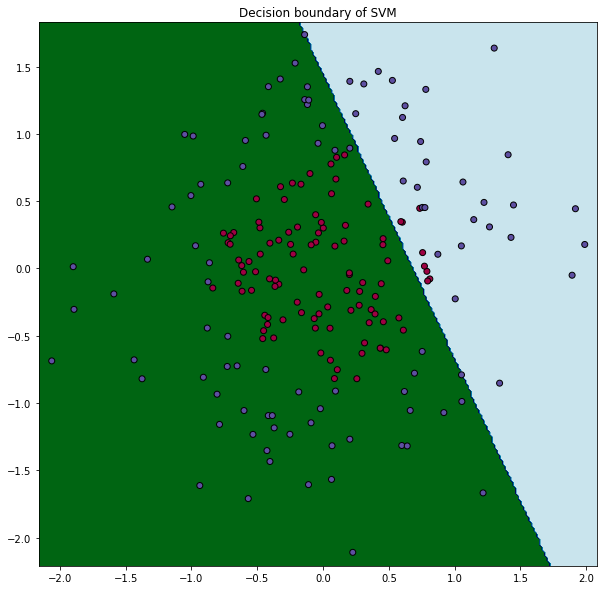Blaaaeh! Linear SVM does horrible here! And the green blinds my eyes. In fact, the data was generated from a bivariate Gaussian distribution, separated by a sphere centered at the origin.

Instead, we can do a Kernel trick. I'm going to it in two ways. First I will turn into Harry Potter and say "Expelliarmus....Wingardium Leviosa" to conjure the following Kernel:

$$K(\bs{x}, \bs{y}) = x_1^2 y_1^2 + x_2^2 y_2^2 + \norm{\bs{x}}\norm{\bs{y}}$$

I doubt that Harry Potter understands support vector machine. Maybe Hermione does. But I will change to Dumbledore, and implement this in sklearn as the following.

In :
# Define a custom function.
def my_kernel(x, y):
a = np.dot(x**2, (y**2).T)
b = np.linalg.norm(x)*np.linalg.norm(y)
return a+b


Next we fit a support vector machine using my_kernel as the custom kernel.

In :
# we create an instance of SVM and fit out data.
clf2 = SVC(kernel=my_kernel, C=1000)
clf2.fit(data, label)

Out:
SVC(C=1000, cache_size=200, class_weight=None, coef0=0.0,
decision_function_shape='ovr', degree=3, gamma='auto',
kernel=<function my_kernel at 0x1a190038c8>, max_iter=-1,
probability=False, random_state=None, shrinking=True, tol=0.001,
verbose=False)

Let's plot the decision boundaries to see how well the classifier does.

In :
plot_decision_boundary(clf2, data, label)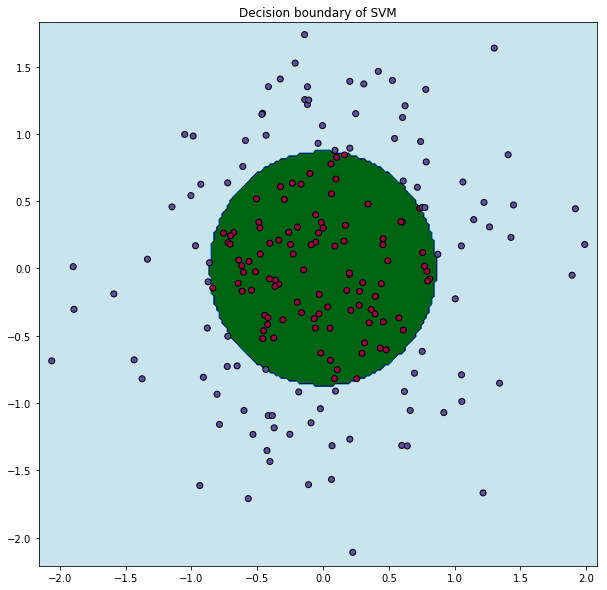This certainly feels like "magic"! What is happening here is that by using a custom kernel, we are actually learning in a three dimension feature space. One can easily show that

\begin{aligned} K(\bs{x}, \bs{y}) &= x_1^2 y_1^2 + x_2^2 y_2^2 + \norm{\bs{x}}\norm{\bs{y}} \\ &=\left<\left(x_1^2, x_2^2, \sqrt{x_1^2+x_2^2}\right)', \left(y_1^2, y_2^2, \sqrt{y_1^2+y_2^2}\right)'\right> \end{aligned}

Hence, simply by the usual dot product in 2D with this custom kernel, it is as if we are optimizing over a 3D space! The advantage of the kernel trick over specifically defining the mapping and learn from a higher dimensional space using a linear kernel is that it is computationally cheaper to use the kernel trick. Calculating the high-dimensional $\phi(\bs{x})$ and then computing the dot product requires $O(p^2)$ time, where $p$ is the number of features. On the other hand, computing the kernel directly only requires $O(p)$ time.

Since for this contrived example here, it is not too expensive to compute $\phi(\bs{x})$, so let's do it here.

In :
# Expand 2D to 3D
X1 = data[:,0]**2
X2 = data[:,1]**2
X3 = np.sqrt(data[:,0]**2+data[:,1]**2)
X = np.vstack([X1, X2, X3]).T
y = label
print("New data has dimension {}.".format(X.shape))

New data has dimension (200, 3).


Let's fit a linear SVM to the new data.

In :
clf3 = SVC(kernel="linear", C=1000)
clf3.fit(X, y)

Out:
SVC(C=1000, cache_size=200, class_weight=None, coef0=0.0,
decision_function_shape='ovr', degree=3, gamma='auto', kernel='linear',
max_iter=-1, probability=False, random_state=None, shrinking=True,
tol=0.001, verbose=False)
In :
from mpl_toolkits.mplot3d import Axes3D

fig = plt.figure(figsize=[10, 10])

# Plotting the new data in 3D
ax.scatter(X[:,0], X[:,1], X[:,2], c=y, s=40, cmap=plt.cm.Spectral)

# obtain the current figure's info and limits
xlim = ax.get_xlim()
ylim = ax.get_ylim()

# create meshgrid for the decision boundary
xx = np.linspace(xlim-1, xlim-2, 100)
yy = np.linspace(ylim-1, ylim-2, 100)
XX, YY = np.meshgrid(xx, yy)

# Plotting the linear decision boundary
C, I = clf3.coef_.ravel(), clf3.intercept_.ravel()
Z = (-C*XX -C*YY - I)/C
ax.plot_wireframe(XX, YY, Z, rstride=10, cstride=10, cmap=plt.cm.Spectral)

Out:
<mpl_toolkits.mplot3d.art3d.Line3DCollection at 0x1a222cd780>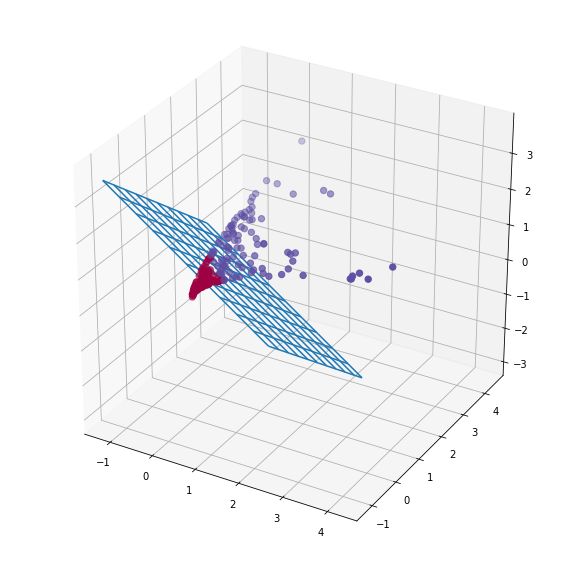This looks great!

For this contrived example, we expanded only one additional dimension. In reality, one of the most popular kernels is called the Gaussian kernel, which uses the radial basis function as its kernel:

$$K(\bs{x}, \bs{y}) = \text{exp}\left( -\frac{\norm{\bs{x}-\bs{y}}^2}{2\sigma^2}\right)$$

This actually corresponds to a mapping from the feature space to an infinite dimensional vector space!

## None-separable Case¶

Finally, we deal with cases when the data is not linear separable (even after using the kernel trick). Also maximum margin method is susceptible to outliers. If we simply add one outlier as the support vector, the decision boundary changes abruptly with it. But do not worry! To fix these two we simply need to slightly modify our algorithm by adding a regularization term. That is, our primal optimization problem becomes

\begin{aligned} \min_{\bs{w}, b} & \quad \frac{1}{2}\norm{\bs{w}}^2 + C\sum_{i=1}^m \xi_i\\ \text{s.t.} & \quad y^{(i)}(\bs{w}\tr \bs{x}^{(i)}+b) \geq 1- \xi_i, \,\, i=1,...,m \\ & \quad \xi_i \geq 0, i=1,...,m. \end{aligned} \tag{6}

Now, we are permitting the functional margin $\beta$ to be less than 1. However, the model imposes a penalty of $C\xi_i$ of weights equal to $1-\xi_i$, so the parameter $C$ controls the degree of regularization. (Recall that earlier I set $C=1000$ in order to ignore regularization.) Wow, this has been a long post! I'm tired, so let's wrap it up with a picture, which is worth 1000 words.

In :
plt.figure(figsize=[10, 10])

# gereate data
X2, y2 = make_blobs(n_samples=100, centers=2, cluster_std = 1,  n_features=2, random_state=0)
plt.scatter(X2[:,0], X2[:,1], c=y2, cmap=plt.cm.coolwarm)

# fit SVM linear model, with various regularization effects
clf_1 = SVC(kernel='linear', C=1000).fit(X2,y2)
clf_2 = SVC(kernel='linear', C=0.1).fit(X2,y2)

# obtain the current figure's info and limits
ax = plt.gca()
xlim = ax.get_xlim()
ylim = ax.get_ylim()

# create meshgrid and obtain the distance from each point to the decision boundary
xx = np.linspace(xlim, xlim, 30)
yy = np.linspace(ylim, ylim, 30)
YY, XX = np.meshgrid(yy, xx)
xy = np.vstack([XX.ravel(), YY.ravel()]).T
Z_1 = clf_1.decision_function(xy).reshape(XX.shape)
Z_2 = clf_2.decision_function(xy).reshape(XX.shape)

# plot decision boundary and margins
ax.contour(XX, YY, Z_1, colors='b', levels=[-1, 0, 1], alpha=0.5, linestyles=['--', '-', '--'])
ax.contour(XX, YY, Z_2, colors='r', levels=[-1, 0, 1], alpha=0.5, linestyles=['--', '-', '--'])

ax.annotate('With Regularization', (3, 4.3), color='r')
ax.annotate('Without Regularization', (-1.2, 2.5), color='b')

# plot support vectors
ax.scatter(clf_1.support_vectors_[:, 0], clf_1.support_vectors_[:, 1], s=300, alpha=0.2, color='red', label = "not regularized")
ax.scatter(clf_2.support_vectors_[:, 0], clf_2.support_vectors_[:, 1], s=300, alpha=0.2, color='black', label = "regularized")

plt.legend()

Out:
<matplotlib.legend.Legend at 0x1a22271780>